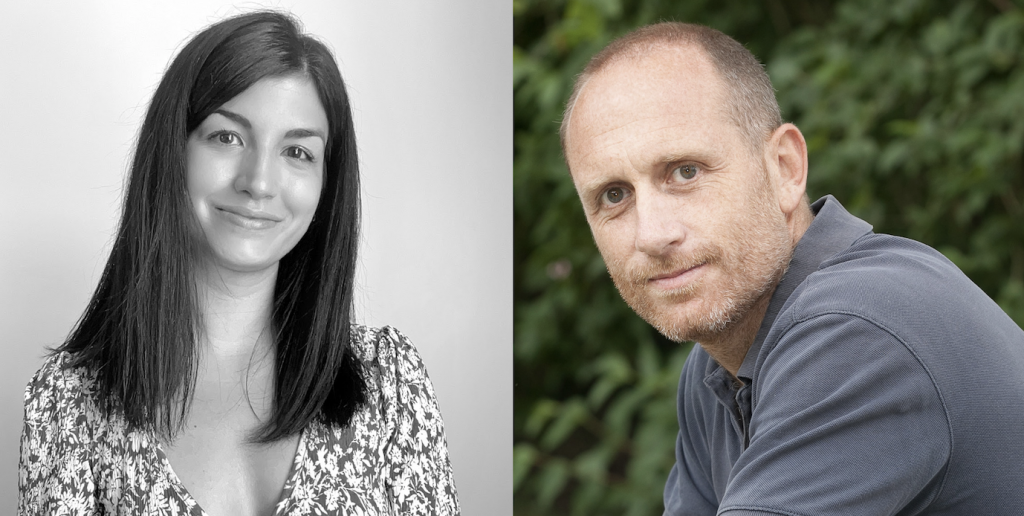## BCAM members gave two different proofs for mathematical conjecture• Javier Fernández De Bobadilla and Irma Pallarés have published one paper in the Journal of the European Mathematical Society and other that one is on revision that present two different proofs for a conjecture in singularities

Researcher from Basque Center for Applied Mathematics – BCAM, Javier Fernández de Bobadilla, Ikerbasque researcher at BCAM, and Irma Pallarés, former PhD at BCAM, given two proofs (the second together with M. Saito, at RIMS, Tokyo) of the Brasselet-Schürmann-Yokura conjecture, an important conjecture in characteristic classes of singular varieties. The background and importance of the conjecture may be explained as follows.

Smooth and singular geometric shapes

Geometry is one of the oldest areas of mathematics, motivated by modelling the physical world. One part of the geometry studies smooth shapes, such as smooth curves, like the circle, or smooth surfaces, like the sphere, but also higher dimensional objects. Despite the smooth manifolds arise in the nature in a significant manner, another phenomenon also appears unavoidably and ubiquitously. For example, there are places in the universe where our laws of physics simply break down, as at centers of black holes and at the beginning of the Big Bang, these special places are called singularities. In mathematics, when singular points appear in geometric objects we call it singular varieties, that is, geometric shapes containing such special points. The geometry of smooth and singular spaces is crucial for the advancement of natural sciences. For instance, smooth and singular manifolds were essential in Einstein’s general relatively theory, but also important in quantum field theory or the geodesy of the Earth.

A brief historical framework of the conjecture

Curvature of curves, surfaces and higher dimensional spaces as our universe has been studied since the 19th century. A landmark theorem, proved in 1848 by Gauss and Bonnet, is a formula relating integral of the curvature of a space with a classical number associated with it called the Euler characteristic. The importance of this formula is that a difficult integral comprising rigid data like curvature is expressed in terms of the Euler characteristic, which is easily computable, and only depends on the shape of the object up to flexible deformations. For example, the Euler characteristic of a polyhedron is just the number of vertices minus number of edges plus the number of faces, and for a surface like doughnuts with several holes, it is related to the number of holes. Curvature belongs to the rigid world of metric geometry and Euler characteristic to the flexible world of topology. Topology is a branch of mathematics that studies the properties that are preserved through deformations, twists and stretches of geometric objects.

Many theorems were proved in the same spirit, for instance in 1865, Riemann and Roch proved a formula relating the complex analysis of certain smooth surfaces with their Euler characteristic. Again, the Riemann-Roch theorem relates information of two very different natures, the topological and the analytical, the former being in the above sense more flexible and computable than the latter. The advent of new tools in topology, provided by the theory of “Characteristic Classes”, introduced by Stiefel and Whitney in the 20th century allowed a huge generalization of results as above. Crowning achievements were the proof of Hirzebruch of the general Riemann-Roch theorem for any dimensions, which unified and generalized in a single formula all the previous results, and the Atiyah-Singer index theorem, one of the most important achievements in the 20th century (it earned Atiyah the Fields medal in 1966), which produced extensions of these results allowing to understand properties of the solutions of partial differential equations in terms of topology. The applications of these two theorems are huge: it should be noted that partial differential equations are essential for mathematical modelling of every kind of phenomena.

Despite importance of spaces with singularities, it was not until 1974, that contributions of many mathematicians provided generalizations to singular spaces of some the results mentioned above. It was not until around 15 years ago that Brasselet, Schürmann and Yokura proved a unification theory for singular varieties similar to the one that Hirzebruch gave in the smooth case.

But Brasselet-Schurmann-Yokura generalization left pending an open conjecture which is crucial for an important type of applications. In 2020-2021, in two papers, J. Fernandez de Bobadilla and I. Pallarés Torres (the second with M. Saito) gave two different proofs of the conjecture. The first paper is on revision and the second accepted in the Journal of the European Mathematical Society.

Pure Mathematics

Throughout the history of science, it has been shown how pure mathematics has been essential for the advancement of other scientific areas, as well as for its application in everyday life. However, pure mathematics is not purposely developed to solve a particular applied problem. The success of its usefulness is due to the interest of researchers to explore a pure area of mathematics for the simple fact of wanting to understand it, and from there mathematical results emerge that are waiting to be applied by future generations.